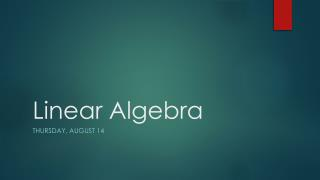DownloadDownload PresentationLinear Algebra

# Linear Algebra

Télécharger la présentation## Linear Algebra

- - - - - - - - - - - - - - - - - - - - - - - - - - - E N D - - - - - - - - - - - - - - - - - - - - - - - - - - -
##### Presentation Transcript

1. Linear Algebra Thursday, august 14

2. Learning Target I will understand what is meant by turn or rotational symmetry and how each point in a figure is related to its image under transformation by rotation.

3. Rotational Symmetry • Rotational symmetry: A figure or design has rotational symmetry if it can be rotated less than a full turn about a point to a position in which it looks the same as the original. The design below has rotational symmetry with its center as the center of rotation and a 60o angle of rotation. This means that it can be rotated 60o, or any multiple of 60o, about its center point to produce an image that matches exactly with the original.

4. Rotational Symmetry • Rotation: A transformation that turns a figure counterclockwise about a point. Polygon A’B’C’D’ below is the image of polygon ABCD under a 60o rotation about point P. If you drew a segment from a point on polygon ABCD to point P and another segment from the point’s image to point P, the segments would be the same length and they would form a 60o angle The line segment D’ to P and D to P are of equal length. The angle formed is 60o

5. Rotational Symmetry • Center of rotation: A fixed point about which a figure rotates. Center of rotation

6. Rotational Symmetry • Angle of rotation: The number of degrees that a figure rotates. In the example below the angle of rotation is 90o. ABC is rotated counterclockwise 90o about point P to result in image A’B’C’.

7. 1.2 In a Spin What angle of rotation would rotate one of the triangles so that the image helps to make this symmetric design? How many copies do you need to make? What is the basic design element? Is there a different basic design element that you could copy?

8. 1.2 In a Spin How is each point X related to its image point X’, the center of rotation O, and the angle of rotation? • OX = OX’ • The measure of angle XOX’ is equal to the angle of rotation

9. 1.2 In a Spin The rotation is 90 degrees. You are not actually making drawings, but you can imagine A “moving” to location G. Can you trace that with your finger?

10. a. The point to image matches are: A to G G to E E to C C to A H to F F to D D to B B to H 1.2 In a Spin AO to GO; HO to FO

11. 3. Each point moves along an arc of a circle with center at O. The radius of the circle is the constant distance between O points A, G, E, and C. Likewise points H, F, D and B move along the arc of a circle with the same center but a different radius 1.2 In a Spin 4. Points X, X’ and the center of the compass star O determine a right angle <XPX’ and line segments XP and X’P have the same length.

12. 1.2 In a Spin 5. This compass star has reflectional symmetry about any of the line segments drawn through the center point O.

13. 1.2 In a Spin For the 120o moves, how many design elements will there be? How many for the 90o move? What will you use for the center of rotation?

14. 1.2 In a Spin

15. C. To create a design with reflectional symmetry you need: • A basic design element • Line of reflection • To create a figure with rotational symmetry you can choose: • Any basic design element • Any center of rotation and • Any angle of rotation • The number of images needed to complete the symmetric design is different for each • For reflectional symmetry you only need the original and a copy • For rotational symmetry, the angle of rotation will determine how many you will need to complete the design 1.2 In a Spin

16. 1.2 In a Spin • Properties of rotational symmetry • A rotation of d degrees about a point P matches any point X on a figure to an image point X’ so that • XP = X’P • The measure of angle XPX’ = d

17. Rate your understanding Learning Target I will understand what is meant by turn or rotational symmetry and how each point in a figure is related to its image under transformation by rotation.

18. Homework tonight • Complete ACE Questions #10, #21, #24 starting on page 18 of BPW